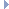# OpenGraph DrawingFramework

v. 2022.02 (Dogwood)

ogdf::steiner_tree::SaveDynamic< T > Class Template Reference

Dynamically updatable weighted Tree for determining save edges via LCA computation. More...

#include <ogdf/graphalg/steiner_tree/SaveDynamic.h>Inheritance diagram for ogdf::steiner_tree::SaveDynamic< T >:

## Public Member Functions

SaveDynamic (EdgeWeightedGraphCopy< T > &steinerTree)
Builds a weighted binary tree based on the given terminal spanning tree. More...

virtual ~SaveDynamic ()

virtual T gain (node u, node v, node w) const
Returns the gain (sum of save edge costs) of the given triple, calculated by an LCA query. More...

virtual edge saveEdge (node u, node v) const
Determines the save edge between two nodes by a LCA query. More...

virtual T saveWeight (node u, node v) const
Determines the weight of the save edge between two nodes by a LCA query. More...

virtual void update (const Triple< T > &t)
Updates the weighted tree data structure given a contracted triple. More...Public Member Functions inherited from ogdf::steiner_tree::Save< T >
Save ()

virtual ~Save ()

## Protected Member Functions

node lca (node u, node v) const
Returns the node in m_tree that is the LCA of two nodes. More...

weight (edge e) const
Returns the weight of an edge in the terminal tree or 0. More...

weight (node v) const
Returns the associated weight of a node v in m_tree, or 0 if it is not associated. More...

## Private Attributes

NodeArray< nodem_cTerminals
Connects terminal nodes in the terminal spanning tree to their leafs in the weighted tree. More...

LCAm_lca
Data structure for calculating the LCAs. More...

node m_root
The root node of the weighted binary tree. More...

const EdgeWeightedGraphCopy< T > * m_steinerTree
The underlying terminal spanning tree this weighted tree instance represents. More...

Graph m_tree
The weighted binary tree to represent the edge weight hierarchy. More...

NodeArray< edgem_treeEdge
Maps each inner node of m_tree to an edge in m_steinerTree. More...

## Detailed Description

### template<typename T> class ogdf::steiner_tree::SaveDynamic< T >

Dynamically updatable weighted Tree for determining save edges via LCA computation.

Note that in this dynamic approach, only the auxiliary tree is updated and not the actual terminal spanning tree (if OGDF_SAVEDYNAMIC_CHANGE_TST is not set).

Definition at line 50 of file SaveDynamic.h.

## ◆ SaveDynamic()

template<typename T >
 ogdf::steiner_tree::SaveDynamic< T >::SaveDynamic ( EdgeWeightedGraphCopy< T > & steinerTree )
inlineexplicit

Builds a weighted binary tree based on the given terminal spanning tree.

Additionally the LCA data structure is initialized.

Parameters
 steinerTree the given terminal spanning tree

Definition at line 58 of file SaveDynamic.h.

## ◆ ~SaveDynamic()

template<typename T >
 virtual ogdf::steiner_tree::SaveDynamic< T >::~SaveDynamic ( )
inlinevirtual

Definition at line 69 of file SaveDynamic.h.

## ◆ gain()

template<typename T >
 virtual T ogdf::steiner_tree::SaveDynamic< T >::gain ( node u, node v, node w ) const
inlinevirtual

Returns the gain (sum of save edge costs) of the given triple, calculated by an LCA query.

Parameters
 u First terminal v Second terminal w Third terminal
Returns
The gain (sum of save edge costs) of the given triple

Implements ogdf::steiner_tree::Save< T >.

Definition at line 82 of file SaveDynamic.h.

## ◆ lca()

template<typename T >
 node ogdf::steiner_tree::SaveDynamic< T >::lca ( node u, node v ) const
inlineprotected

Returns the node in m_tree that is the LCA of two nodes.

Parameters
 u first node v second node
Returns
the LCA of u and v

Definition at line 251 of file SaveDynamic.h.

## ◆ saveEdge()

template<typename T >
 virtual edge ogdf::steiner_tree::SaveDynamic< T >::saveEdge ( node u, node v ) const
inlinevirtual

Determines the save edge between two nodes by a LCA query.

Parameters
 u First node v First node
Returns
The save edge between two given nodes

Implements ogdf::steiner_tree::Save< T >.

Definition at line 111 of file SaveDynamic.h.

## ◆ saveWeight()

template<typename T >
 virtual T ogdf::steiner_tree::SaveDynamic< T >::saveWeight ( node u, node v ) const
inlinevirtual

Determines the weight of the save edge between two nodes by a LCA query.

Parameters
 u First node v First node
Returns
Weight of the save edge between two given nodes

Implements ogdf::steiner_tree::Save< T >.

Definition at line 99 of file SaveDynamic.h.

## ◆ update()

template<typename T >
 virtual void ogdf::steiner_tree::SaveDynamic< T >::update ( const Triple< T > & t )
inlinevirtual

Updates the weighted tree data structure given a contracted triple.

The update of the weighted tree is performed dynamically. To achieve this, the weighted tree is traversed bottom-up, starting at the three leaves corresponding to the terminals in the triple. It takes time O(height of m_tree).

Parameters
 t The contracted triple

Implements ogdf::steiner_tree::Save< T >.

Definition at line 128 of file SaveDynamic.h.

## ◆ weight() [1/2]

template<typename T >
 T ogdf::steiner_tree::SaveDynamic< T >::weight ( edge e ) const
inlineprotected

Returns the weight of an edge in the terminal tree or 0.

Definition at line 259 of file SaveDynamic.h.

## ◆ weight() [2/2]

template<typename T >
 T ogdf::steiner_tree::SaveDynamic< T >::weight ( node v ) const
inlineprotected

Returns the associated weight of a node v in m_tree, or 0 if it is not associated.

Definition at line 265 of file SaveDynamic.h.

## ◆ m_cTerminals

template<typename T >
 NodeArray ogdf::steiner_tree::SaveDynamic< T >::m_cTerminals
private

Connects terminal nodes in the terminal spanning tree to their leafs in the weighted tree.

Definition at line 278 of file SaveDynamic.h.

## ◆ m_lca

template<typename T >
 LCA* ogdf::steiner_tree::SaveDynamic< T >::m_lca
private

Data structure for calculating the LCAs.

Definition at line 279 of file SaveDynamic.h.

## ◆ m_root

template<typename T >
 node ogdf::steiner_tree::SaveDynamic< T >::m_root
private

The root node of the weighted binary tree.

Definition at line 273 of file SaveDynamic.h.

## ◆ m_steinerTree

template<typename T >
 const EdgeWeightedGraphCopy* ogdf::steiner_tree::SaveDynamic< T >::m_steinerTree
private

The underlying terminal spanning tree this weighted tree instance represents.

Definition at line 277 of file SaveDynamic.h.

## ◆ m_tree

template<typename T >
 Graph ogdf::steiner_tree::SaveDynamic< T >::m_tree
private

The weighted binary tree to represent the edge weight hierarchy.

Definition at line 271 of file SaveDynamic.h.

## ◆ m_treeEdge

template<typename T >
 NodeArray ogdf::steiner_tree::SaveDynamic< T >::m_treeEdge
private

Maps each inner node of m_tree to an edge in m_steinerTree.

Definition at line 272 of file SaveDynamic.h.

The documentation for this class was generated from the following file: